# Automation of plotting long-term records

Hi
I would like to control the joulescope headless and record for 1h and save a plot as png.
Tried to make use of pyjoulescope_examples

Proof of concept:
\$ python bin\capture.py --duration 60 --jls 1 test_60s_capture_v1.jls

\$ python bin\current_range_extract.py test_60s_capture_v1.jls --plot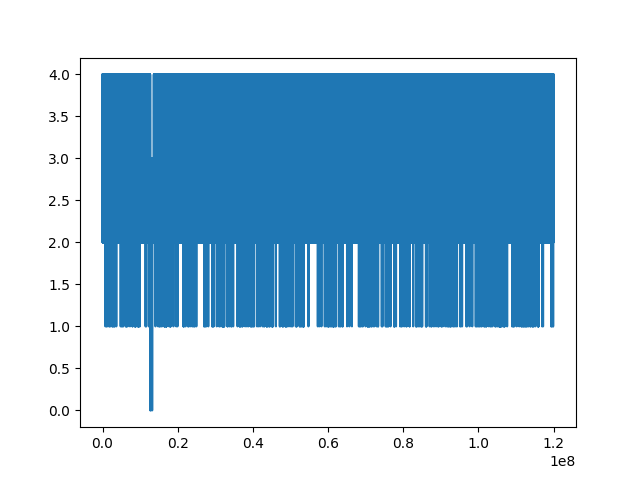but the result is not as good as the ui version printscreen

I would like to have the statistics on the plot too as in the ui and perhabs exportet the stats to a file.
BR

Lukas

Hi @lukGWF and welcome to the Joulescope forum!

It sounds like you already know how to make automated captures. If I understand correctly, you are now trying to open a JLS and plot it to a PNG file. The Joulescope UI does not support this as an automated method, only by using the Joulescope UI application.

You can find the Joulescope UI waveform plotting code in joulescope_ui.widgets.waveform, starting with waveform.py. You could modify this code to use it directly, but that is not something we currently support.

Note that the `current_range_extract` script plots the current range selection, not actually the current. I am assuming that you want to plot the current.

One key difference between your Matplotlib plot and the Joulescope UI plot is the concept of “reductions”. Instead of plotting every point, the Joulescope UI plots the mean of all samples represented by a pixel. It also can plot min & max, but I see you have that turned off. Instead of using `DataReader.samples_get`, you can call `DataReader.data_get`. For example, if you want to plot 1000 points, you can do something like:

``````from joulescope.data_recorder import DataReader
start_idx, stop_idx = r.sample_id_range
incr = (stop_idx - start_idx) // 1000
data = r.data_get(start_idx, stop_idx, incr, units='samples')
i = data[:,0]
``````

Now, you ideally want to specify `incr` so that it exactly matches the number of x-axis pixels in your PNG plot. However, Matplotlib will do mostly the right thing if you provide too many or too few pixels.

Does this help?

Hi @mliberty

``````#!/usr/bin/env python3
# -*- coding: utf-8 -*-
from joulescope import scan_require_one
import sys
import argparse
import queue
import signal
from PySide2 import QtCore, QtGui, QtWidgets
from joulescope_ui.main import MainWindow
from joulescope_ui.command_processor import CommandProcessor
from joulescope_ui.preferences_def import preferences_def
import joulescope_ui.widgets.waveform.waveform
from joulescope_ui.recording_viewer_factory import factory
import joulescope_ui.main
import joulescope_ui.entry_points.ui

from joulescope.units import three_sig_figs

def testrunAndShowAndSaveDirectly():
filename = "test.jls" # a 30s capture

#rc = joulescope_ui.main.run(None, None, None, filename, None)
app = QtWidgets.QApplication(sys.argv)
cmdp = CommandProcessor()
cmdp = preferences_def(cmdp)
ui = MainWindow(app, None, cmdp, None)
wv = joulescope_ui.widgets.waveform.WaveformWidget(ui, cmdp, None)

from joulescope_ui.main import run

png = wv._export_as_image()
png.save("df.png")
``````

I do get the picture but it is only a snipped of the screen.
BR
Lukas

Qt is very picky about Widget layout. With the approach above, the `wv` widget is not part of the layout, so it will not behave correctly. Also, the code is going to load the last UI settings, which means anytime you use the actual Joulescope UI it may affect this script. It also never executes the Qt event loop, so Qt will never render.

I see three possible approaches:

1. Attempt to use most of the full Joulescope UI code but load “fixed” defaults.
2. Create a stripped-down Qt application with just the WaveformWidget.
3. Use Matplotlib

(1) and (2) are going to be challenging. I took a quick look, and I don’t see any quick path to get this done. Unfortunately, we don’t have the resources to develop either (1) or (2) in the near future.

(3) is relatively easy. Here is some code that may be good enough:

``````#!/usr/bin/env python3
# -*- coding: utf-8 -*-

import argparse
import numpy as np
import sys
import matplotlib.pyplot as plt
from joulescope.view import data_array_to_update

def get_parser():
p = argparse.ArgumentParser(
description='Convert a JLS file to an image plot.')
help='The input filename path.')
help='The output filename path.')
type=int,
default=1000,
help='The number of samples to display')
return p

def run():
args = get_parser().parse_args()
start_idx, stop_idx = r.sample_id_range
d_idx = stop_idx - start_idx
print(f'{start_idx}:{stop_idx} ~ {d_idx}')
f = r.sampling_frequency
incr = d_idx // args.sample_count
data = r.data_get(start_idx, stop_idx, incr, units='samples')

x = np.linspace(0.0, d_idx / f, len(data), dtype=np.float64)
x_limits = [x, x[-1]]
s = data_array_to_update(x_limits, x, data)

f = plt.figure()
ax_i.grid(True)
ax_i.plot(x, s['signals']['current']['µ']['value'])
ax_i.set_xlabel('Time (seconds)')
ax_i.set_ylabel('Current (A)')
# plt.show()
f.savefig(args.output)

if __name__ == '__main__':
sys.exit(run())
``````

What do you think?

I did more testing and edited the above code. It now works correctly on a bunch of JLS files and produces plots like this: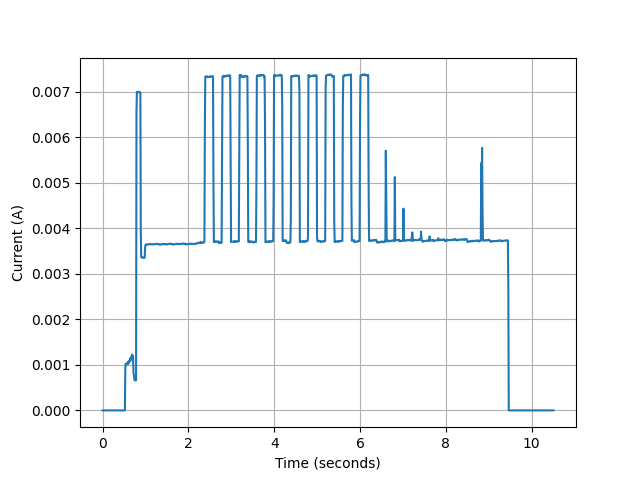Hi
That is fast!

I am not familiar with matplotlib that’s why I try to get the other up and running.

I would need to add the stats to continue. If you could help me on that too I would be glad.

When I tested with another data the input data was mirrored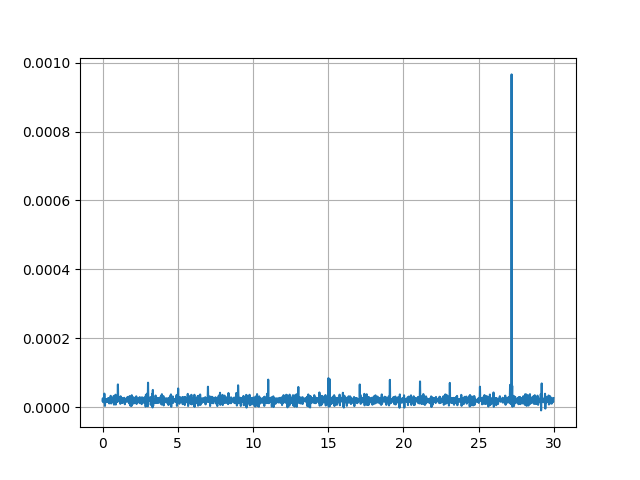the axis are correct but the peak should be on the left side.

I updated the code to display statistics and added it to pyjoulescope_examples here. The plot now looks like: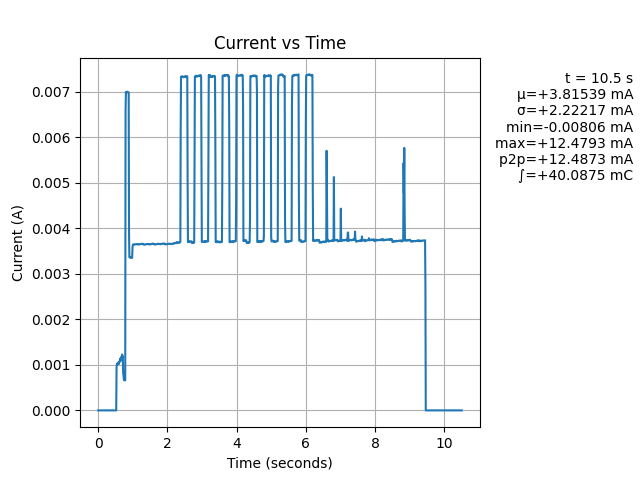Here is the command line I used:

``````python bin\jls_plot.py e:\evk.jls --out out.png --stats
``````

I took a look at a few JLS files, and they look right without any mirroring. Could you please open that same JLS file in the Joulescope UI to confirm that it is mirrored?

Checked a 42s plot regarding mirroring
matplotlib variant here: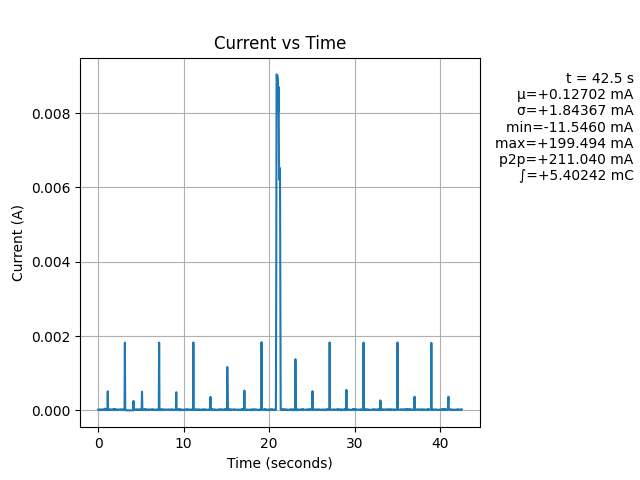and the joulescope variant:

I think that they are the same. Note that the `jls_plot` code is not plotting min/max. I followed what you had in your initial plot with min/max turned off. In the Joulescope UI, turn off min/max and see if they look the same. Note that adding min/max to the `jls_plot` script is easy.

Hi
You are right. When removing the min/max it is the same.

Can you add the charge also to the stat?

Thanks for that support!!!

Hi @lukGWF - The ∫ (integral) shown in the image above displays the integral of current over the window, which is the charge. The units are in coulombs (C), which is equivalent to ampere seconds. If you want ampere hours (Ah), divide by 3600.

Hi
I have continued and am finding peaks in long-time records. I have optimized the samplerate (recording) and --sample_count to a speedup the process of analysation. Using 200k samplerate and sample_count of: 500 the elapsed time is 10s, at 50:93s, at 5:286s.
When I look at htop the process only used one single cpu. Do you think it is possible to make use of multiprocessing? The time consuming loop is the data_recorder.py (v0.9.7) line 1061… “_statistics_get_handler_float32_v2”.
The RAM consumption is 2GB.
One CPU-core is at 100%.
What do you expect could be improved in time using change the package to cupy for these basic operations?

Hi @lukGWF

If I understand correctly, you are profiling the performance of `jls_plot.py`. The JLS v1 file format that you are using offers decent performance on huge files, but our newer JLS v2 file format is much, much faster. You can find details on JLS v2 on GitHub. The Joulescope UI can already display JLS v2, but it still records to v1.

If you want, you can provide `--jls 2` to `capture.py`, and it will record a JLS v2 file.

The next challenge is reading the JLS v2 file, as we do not have many examples yet. However, I just updated the jls_plot.py example to also handle JLS v2 files.

Does this work for you?

Hi @mliberty
Until now I was recording up to ~20GB in one file and the script was working.
Now I did 3600s which allocated 28GB and then the output of the scripts was

2022-02-10 16:46:25,157 - main - INFO - Reading JLS v1: C:\code\pyjoulescope_examples_\20220209_standby_jls2.jls
Traceback (most recent call last):
File “C:\code\pyjoulescope_examples_\bin\jls_plot.py”, line 810, in
sys.exit(run())
File “C:\code\pyjoulescope_examples_\bin\jls_plot.py”, line 384, in run
data = r.data_get(start_idx, stop_idx, incr, units=‘samples’)
File “C:\tools\miniconda3\envs\pyjsui\lib\site-packages\joulescope\data_recorder.py”, line 1047, in data_get
out = stats_array_factory(out_len)
File “joulescope\stream_buffer.pyx”, line 206, in joulescope.stream_buffer.stats_array_factory
numpy.core._exceptions.MemoryError: Unable to allocate 322. GiB for an array with shape (1440003902, 6) and data type [(‘length’, ‘<u8’), (‘mean’, ‘<f8’), (‘variance’, ‘<f8’), (‘min’, ‘<f8’), (‘max’, ‘<f8’)]`

Can you tell me what arguments you are providing to `jls_plot`? I suspect that `sample_count` is way too big. You ideally want `sample_count` to be the width of the plot axes in pixels.

Perhaps a better name for `sample_count` would be `plot_point_count`. It’s the number of x,y pairs that are plotted onto the axes.

Thanks for this hint. I need to give more information to you that you can understand the task. I extended the software to be able to recognize current-patterns as they are defined using different parameters length, charge, heights … I got that working but need the 2MSps resolution. So I also need to be able to load files with that size. What is the max length I can read so I can iterate over the file by loading parts in the lines